Courses

# NCERT Solutions(Part- 1)- Comparing Quantities Class 8 Notes | EduRev

## Class 8 : NCERT Solutions(Part- 1)- Comparing Quantities Class 8 Notes | EduRev

The document NCERT Solutions(Part- 1)- Comparing Quantities Class 8 Notes | EduRev is a part of the Class 8 Course Class 8 Mathematics by VP Classes.
All you need of Class 8 at this link: Class 8

Question: In a primary school, the parents were asked about the number of hours they spend per day in helping their children to do homework. There were 90 parents who helped for 1/2 hour to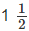hours. The distribution of parents according to the time for which, they said they helped is given in the adjoining figure; 20% helped for more thanhours per day; 30% helped for 1/2 hour tohours; 50% did not help at all.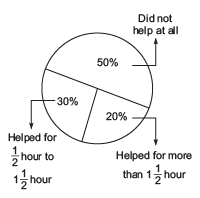Using this, answer the following:

(i) How many parents were surveyed?

(ii) How many said that they did not help?

(iii) How many said that they helped for more thanhours?

Solution:

(i) Since, 30% of total surveyed parents helped their children for "1/2 hours tohours”. And 90 parents helped their children for “ 1/2  hours tohours”.

∴ 30% [surveyed parents] = 90

or 30/100 * [surveyed parents] = 90

or surveyed parents =  90 *100/30

= 3 * 100

= 300

(ii) Since 50% of surveyed parents did not help their children.

∴ Number of parents who did not help = 50% of surveyed parents

= 50% of 300

= 50/100 * 300 = 150

(iii) Since, 20% of the surveyed parents help their children for more thanhours.

i.e. 20% of surveyed parents help for more thanhours.

∴ Number of parents who helped for more thanhours = 20% of 300

= 20/100 * 300

= 20 * 3 = 60

Note: ‘of’ means multiplication.

Exercise 8.1

Question 1. Find the ratio of the following:

(a) Speed of a cycle 15 km per hour to the speed of scooter 30 km per hour.

(b) 5 m to 10 km (c) 50 paise to Rs 5

Solution:

Remember

In a ratio, the quantities are in the same unit. If they are not in the same units, then first we convert them in the same unit.

(a) Speed of cycle = 15 km per hour

Speed of scooter = 30 km per hour

∴ Ratio = Speed of cycle/Speed of scooter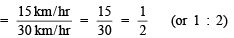(b) Ratio = 5m/10 * 1000m                  (Changing 10 km into m)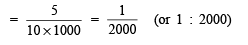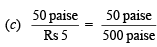(Changing Rs 5 to paise)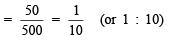Question 2. Convert the following ratios to percentages.

(a) 3 : 4             (b) 2 : 3

Solution:

(a) Q 3 : 4 = 3/4

∴ 3/4 = 3/4 * 100%

= (3 * 25)% = 75%

(b) Q 2 : 3 = 2/3

∴ 2/3 = 2/3 * 100%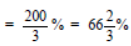Question 3. 72% of 25 students are good in Mathematics. How many are not good in Mathematics?

Solution: ∵ 72% of 25 are good in Mathematics.

∴ (100 – 72)% of 25 students are not good in Mathematics.

or 28% of 25 students are not good in Mathematics

or 28/100 * 25 =7 students are not good in Mathematics.

Question 4. A football team won 10 matches out of the total number of matches they played. If their win percentage was 40, then how many matches did they play in all?

Solution: Number of matches won by the team = 10

∵ The team won 40% of total number of match.

∴ 40% of [Total number of match] = 10

or 40/100 * [Total number of match] = 10

Total number of matches =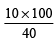=  25

Thus, the total number of match played = 25

Question 5. If Chameli had Rs 600 left after spending 75% of her money, how much did she have in the beginning?

Solution: ∵ Chameli made spending of Rs 75%.

∴ She is left with Rs (100 – 75)% or Rs 25%.

But she is having Rs 600 now.

∴ 25% of total money = Rs 600

or  25/100  of total money = Rs 600

or  Total money =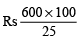= Rs 600 x 4

= Rs 2400

Thus, she had Rs 2400 in the beginning.

Question 6. If 60% people in a city like cricket, 30% like football and the remaining like other games, then what per cent of the people like other games? If the total number of people are 50 lakh, find the exact number who like each type of game.

Solution:

∵ People who like cricket = 60%

People who like football = 30%

∴ People who like other games = [100 – (60 + 30)]%

= [100 – 90]%

= 10%

Now, Total number of people = 50,00,000

∴ 60% of 50,00,000 = 60/100 * 5000000

= 6 * 500000

= 30,00,000

30% of 5000000 = 30/100 * 5000000

= 3 * 500000

= 15,00,000

10% of 5000000 = 10/100 * 5000000

= 1 * 500000

= 5,00,000

Thus, number of people who like

Cricket = 30,00,000

Football = 15,00,000

Other games = 5,00,000

Finding the Increase or Decrease Per Cent (Discounts)

Example. A shirt is marked at Rs 850 and is sold for Rs 765. What is the discount and discount per cent?

Solution:

Marked price of the shirt = Rs 850

Selling price of the shirt = Rs 765

∴ Discount = [Marked price] – [Selling price]

= Rs 850 – Rs 765

= Rs 85

Now, discount per cent = 85/850 * 100%

= 10%

Note: For calculating discount per cent we use the marked (or the list price as the base)

Profit And Loss

[Finding cost price/selling price, Profit %/Loss %]

Remember

I. Total cost price = Cost price + Overhead expenses.

II. Overhead expenses means, the expenses like amount spent on repairs, labour charges, transportation, etc.

III. Profit % = Profit/CP *100 and Loss % = Loss/CP * 100

IV. Profit % or Loss % is always calculated with cost price as the base.

V. Profit = Profit % x CP/100 and Loss = Loss % * CP/100

Example. Sarla purchased a second hand washing machine for Rs 5000 and spent Rs 400 on its repairs. She sold it for Rs 6300. Find here loss or profit per cent.

Solution:

Total cost price = Rs 5000 + Overhead expenses

= RS 5000 + Rs 400 = Rs 5400

Selling price = Rs 6300

∵ Selling price > Cost price

∴ Profit = Rs 6300 – Rs 5400 = Rs 900

or Profit per cent =900/5400 x 100% = 50/3% =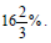Thus, she earned a profit ofSales Tax/Value Added Tax

Taxes are of two types Direct taxes (such as income tax, wealth tax, professional tax, etc.) and indirect taxes (such as excise tax, sales tax, value added tax VAT, service tax, etc.). Direct taxes are collected by the Central Govt. and sales tax or value added tax (VAT) is collected by the State Government. This is charged on the bill amount. Shopkeepers collect it from the customers and deposit to the government.

Example. Rahul bought a TV for Rs 89,600 including a tax of 12%. Find the cost of the TV before VAT was added.

Solution: Let the cost of TV before VAT (or the original cost) = Rs 100

∴ VAT = 12% of the original cost

= 12/100 * Rs 100 = Rs 12

∴ Cost of TV including VAT = Rs 100 + Rs 12 = Rs 112

If price including VAT is Rs 112, then original cost = Rs 100

If price including VAT is Rs 89,600, then original cost = 100/112  x 89,600 = Rs 80,000

Remember

Customers pay VAT to the shopkeeper. Government collects it from the shopkeeper, therefore it cannot be a part of his profit.

Offer running on EduRev: Apply code STAYHOME200 to get INR 200 off on our premium plan EduRev Infinity!

93 docs|16 tests

,

,

,

,

,

,

,

,

,

,

,

,

,

,

,

,

,

,

,

,

,

;